# Laws of Reflection

We know that light is a form of energy which can undergo various phenomena like refraction, reflection, diffraction, and interference. In this session, let us know more about the laws of reflection (first law of reflection and second law of reflection), types of reflection, examples, differences, and total internal reflection.

## What Is Law of Reflection?

Definition:

1. The law of reflection defines that upon reflection from a smooth surface, the angle of the reflected ray is equal to the angle of the incident ray, with respect to the normal to the surface that is to a line perpendicular to the surface at the point of contact.
2. The reflected ray is always in the plane defined by the incident ray and the normal to the surface at the point of contact of the incident ray.

The images produced by plane mirrors and curved mirrors can be understood by the law of reflection.

Law of reflection is defined as:

The principle when the light rays fall on the smooth surface, the angle of reflection is equal to the angle of incidence, also the incident ray, the reflected ray, and the normal to the surface all lie in the same plane.

### What Is Reflection of Light?

The process through which light rays fall on the surface and get bounced back is known as a reflection of light.

## Types of Reflection

### Regular Reflection:

The plane mirrors with a smooth surface produce this type of reflection. In this case, the image is clear and very much visible. The images produced by plane mirrors are always virtual, that is they cannot be collected on a screen.

In the case of curved mirrors with a smooth surface, we can see the images of reflection either virtually or really. That is, the images produced by curved mirrors can be either real (collected on a screen and seen), or virtual (cannot be collected on a screen, but only seen).

### Irregular Reflection:

Unlike mirrors, most natural surfaces are rough on the scale of the wavelength of light, and, as a consequence, parallel incident light rays are reflected in many different directions irregularly, or diffusely. Hence, diffuse reflection helps in seeing the objects and is responsible for the ability to see most illuminated surfaces from any position.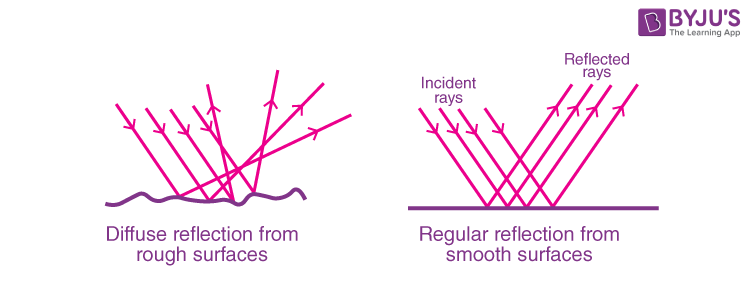In both regular and irregular reflections, the laws of reflection are followed.

## Law of Reflection Formula:

The law of reflection formula is given as:

 θi = θr

Where,

• θis the angle of incidence
• θis the angle of reflection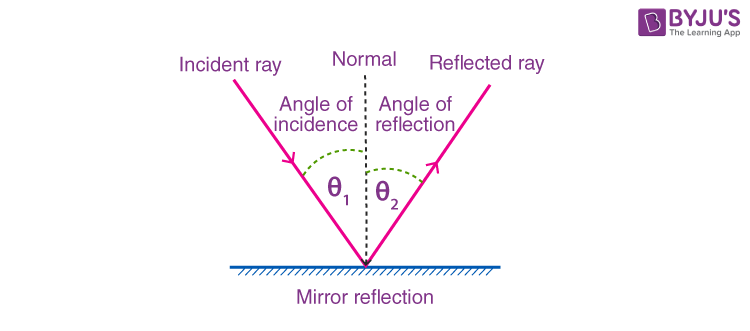### What Is Angle of Reflection?

The angle of reflection 0r of a ray is the angle measured from the reflected ray to the normal surface.

### Calculation of Angle of Incidence and Angle of Reflection

The angle of incidence and the angle of reflection are calculated by drawing a normal line that is perpendicular to the reflecting surface.

## Examples of Laws of Reflection

Q1: A ray of light is incident towards a plane mirror at an angle of 30° with the mirror surface. What will be the angle of reflection?

Ans:

Since the angle of incidence is measured between the incident ray and the normal, so, here the angle of incidence is not  60°

According to the Law of Reflection,

θi = θr

Hence,

Angle of Reflection = 60°

Q2: A light ray strikes a reflective plane surface at an angle of 54° with the surface.

(i) Calculate the angle of incidence.

(ii) Calculate the angle of reflection.

(iii) Calculate the angle made by the reflected ray and the surface.

(iv) Calculate the angle made by the incident and reflected rays.

Ans:

(i) Angle of incidence, θi = 90° – 54°=36°

(ii) Angle of Reflection, θr = 36° (As per Law of Reflection)

(iii) Angle made by the reflected ray and the surface,

90° – θr

(iv) Angle made by the incident and reflected rays,

θi + θ=36° + 36° = 72°

Q3: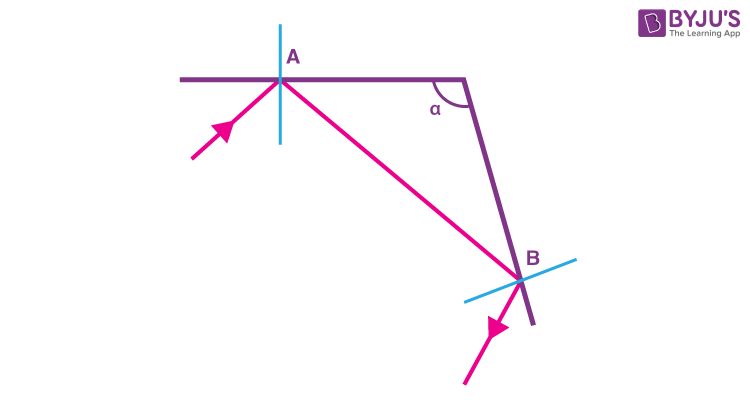Find angle α made by the system of the two mirrors shown in the figure above so that the incident ray at A and the reflected ray at B are parallel.

Ans: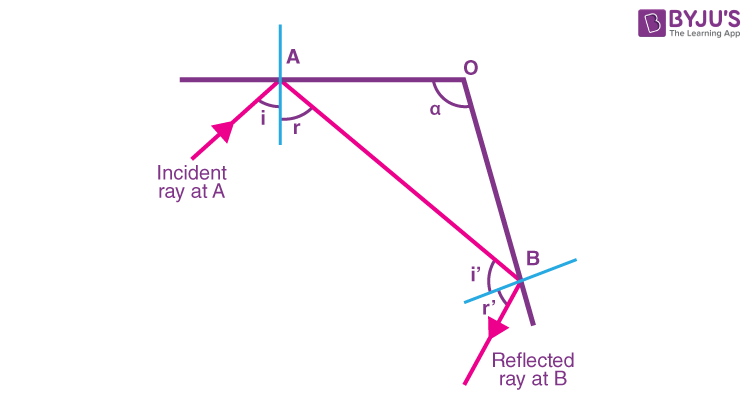We first complete the given diagram with the angles of incidence and reflection as shown above and also label the incident and reflected rays.

For the incident ray at A and the reflected ray at B to be parallel, angles i + r and i’ + r’ have to be supplementary. (geometry: parallel lines cut by a transversal).

Hence,

i + r + i’+ r’ = 180 °

by law of reflection : r = i and r’ = i’

Substitute to obtain

i + i + i’ + i’ = 180 °

i + i’ = 90

In triangle AOB, we have

α + (90 – r) + (90 – i’) = 180 °

α = r + i’ = i + i’ = 90 °

If α = 90 °, the incident ray at A and the reflected ray at B are parallel.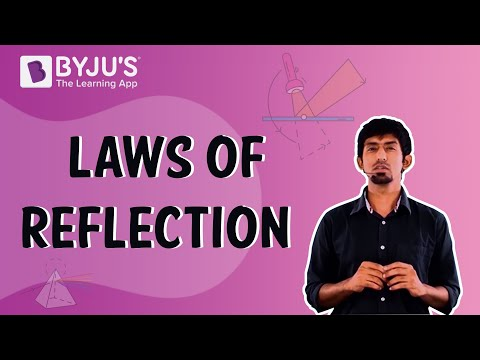## Differences between Regular and Irregular Reflection

 S.No. Regular Reflection Irregular Reflection 1 It occurs when all the reflected rays from a given smooth surface are parallel to the parallel incident rays. It occurs when for a given set of parallel incident rays, the reflected rays do not remain parallel to each other. 2 This occurs from smooth surfaces like a mirror, silver spoon, etc. This occurs from rough surfaces like wood, table, door, book, etc. 3 The image is formed and seen. It helps to see objects. No images or blurry images can be seen.

### Concave Mirrors:

By reflection of light, concave mirrors give real, inverted images if the object is beyond the focus and a virtual, erect, enlarged image if the object has a distance less than the focal length from the pole of the mirror.

Uses of Concave Mirrors:

1. Concave mirrors are used in torches, searchlights, and headlights of vehicles to get powerful parallel beams of light.
2. Concave mirrors are also used as shaving mirrors to see a larger image of the face.
3. Dentists use concave mirrors to see bigger images of the teeth of the patients.
4. Large concave mirrors are used to focus sunlight to produce heat in the solar furnaces.

### Convex Mirrors:

By the reflection of light convex Mirrors always give a virtual, erect, diminished image of the object kept infront of the mirror.

Uses of Convex Mirrors:

1. The convex mirror is used as a side-view mirror in vehicles to give a smaller view of the vehicles coming from behind.
2. They are used in shops and supermarkets and any other place where there is a requirement for detecting burglars.
3. Convex mirrors are used in making lenses for sunglasses.
4. Convex mirrors are used in magnifying glasses, and telescopes.
5. Convex mirrors are used to reflect street light; because they can reflect over a wide area.
6. Convex mirrors are kept at the street corners to avoid collisions.

## Total Internal Reflection

When light passes from a denser medium to a lighter medium at an angle more than the critical angle required for refraction, then the light is reflected back into the denser medium. This is a phenomenon called Total Internal Reflection. The light undergoing the total internal reflection also follows the ordinary laws of reflection for light as shown below: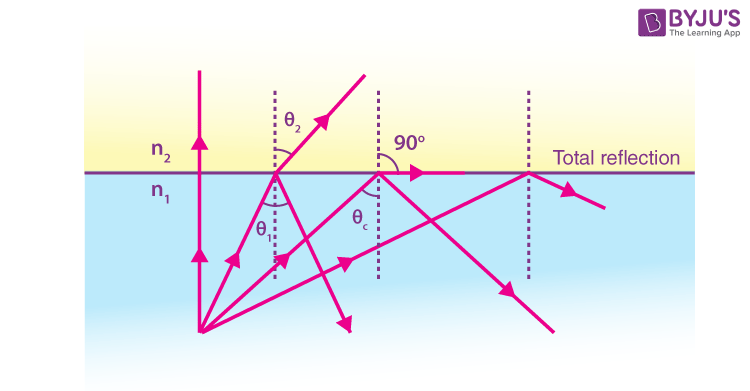The phenomenon, total internal reflection, is taken advantage of fibre in piping light in a curved path. The light directed down a narrow fibre of glass or plastic repeatedly reflects from the fibre-air interface at an angle larger than the critical angle. Optical fibres can transmit light over long distances without any loss of intensity.

Sequences of light pulses are used to transmit information through an optical fibre network with the help of this total internal reflection. Medical instruments like ‘endoscopes’ use the total internal reflection of light through an optical fibre bundle to image internal organs.

## Uses of Reflection

1. Reflection is used in periscopes to view advancing enemies on the battlefield from a safe position.
2. Reflection is the reason why we see objects.
3. Reflection by a concave mirror and a convex mirror has many uses as listed above.
4. Reflection helps in medical diagnosis and optical communications.
5. Light and Sound both follow the law of reflection, both being waves.
6. Using the law of reflection for sound and light, we can measure the distances accurately to objects.
7. Reflection is the reason why we hear the echo of sound.

Related Articles:

## Watch the video and revise all the important concepts in the chapter Light Reflection and Refraction Class 10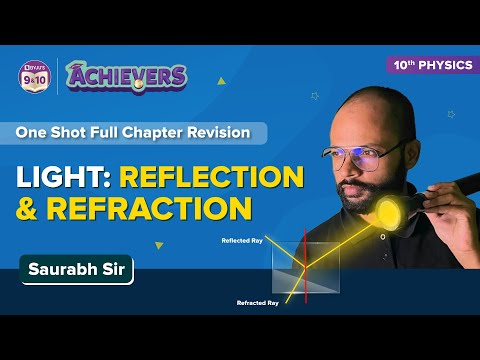## Frequently Asked Questions – FAQs

Q1

### Choose the correct option: What is the dimension of the object as compared to the wavelength of the propagating wave when reflection occurs?a. Largeb. Smallc. Samed. Very small

Correct answer: Option a. Reflection occurs when a propagating electromagnetic wave impinges upon an object which has very large dimensions when compared to the wavelength of the propagation wave. Reflection occurs from the surface of the Earth and from buildings and walls.
Q2

### Choose the correct answer: What is the case of reflection, in the course of the second medium being a perfect dielectric?a. Loss of energy during absorptionb. Total energy reflected back to the first mediumc. No loss of energy in the absorptiond. Total energy transmitted into the second medium

Correct answer: Option c. There is no loss of energy in absorption. If the plane wave is incident on a perfect dielectric, part of the energy is transmitted into the second medium and part of the energy is reflected back into the first medium.

Q3

### State True/False: The angle of incidence is equal to the angle of reflection for perfect reflection.

The given statement is true. For complete wave reflection, the angle of incidence should be the same as the angle of the reflection. In such cases, the reflection coefficient is unity and the transmission coefficient is zero.

Q4

### If a child crawls toward a mirror at the rate of 0.20 m/s, then at what speed the child and the image will come close to each other?

In one second, the child comes closer to the mirror by 0.2 meters. In the same second, the image comes closer to the mirror by 0.2 meters. Now, the relative distance between the child and the image is reduced by 0.4 meters in one second. Hence, the speed the child and the image will come close to each other is 0.4 m/s.
Q5

### State the second law of reflection.

The second law of reflection states that “the incident ray, the normal to the surface at the point of incidence and the reflected ray, all lie in the same plane”.

Hope you learned the first law of reflection and the second law of reflection. To know more, stay tuned with BYJU’S.

Test your knowledge on Laws of reflection